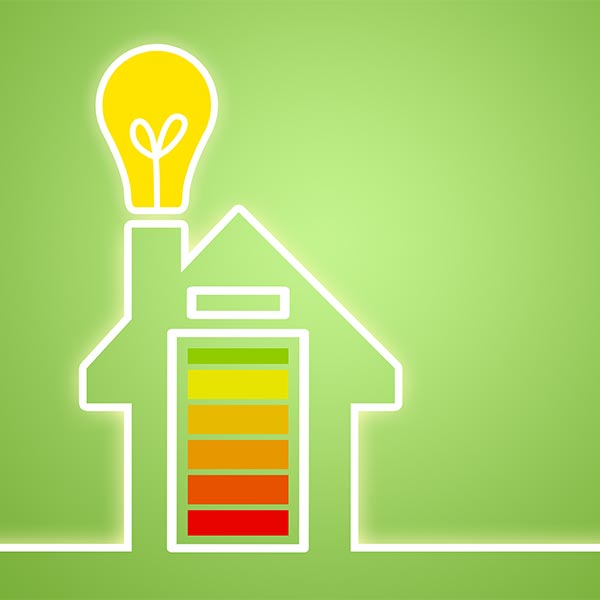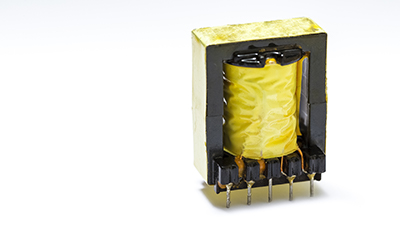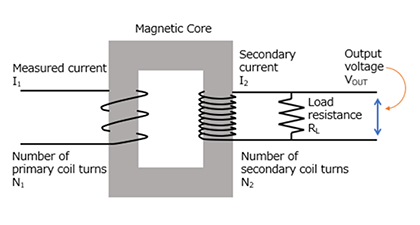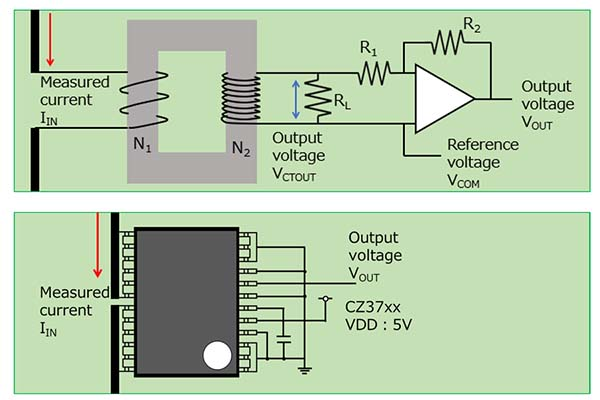# ACCT or Currentier ?

What is ACCT?

How do I replace it?

#01 Mini-lessons on topics about current detection

Current Sensors

One simple current detection method is the use of ACCTs. The key advantage of the ACCT method is its low cost. However, it has the disadvantages of poor accuracy and being incapable of measuring DC currents.
If you would like to add functions but are unable to do so because you cannot measure DC currents, we recommend using a Currentier instead of an ACCT.

## 1. What is ACCT?

ACCT current detection uses a device known as a current transfomer (current transducer), often written simply as "CT". Due to the principles it uses to measure current, this method cannot measure DC current. It is exclusively for measuring AC current, which is why was call it "AC"CT.

[Note]

A current sensor that uses a Hall element and a magnetic core is sometimes called a "DCCT." The principle is the same as that of a current sensor with a core. Please note that the measurement principle used by DCCTs is different from that of ACCTs, introduced here.

ACCTs are often used for current detection in next-generation power device applications using SiC and GaN such as charging station, PAC, UPS.## 2. Why can't the ACCT measure DC current?

Current transfomers are unable to measure DC current because they use the principle of electromagnetic induction.

Figure 1 shows a magnet and a coil.

When the magnet is moved closer to or further away from the coil, the magnetic field in the coil changes. A current flows through the coil to cancel out this change in the magnetic field. This is called the law of electromagnetic induction."Figure 1. Law of electromagnetic induction

Figure 2 shows a structural diagram of a current transfomer.

When the measurement current (AC) flows through the primary side, the magnetic field of the primary coil changes." On the secondary side, due to the law of electromagnetic induction shown in Figure 1, a current flows to cancel out this change in magnetic field. This current is converted to a voltage using a load resistor. This is the principle by which ACCTs operate. DC currents do not cause magnetic field changes, so ACCTs are incapable of measuring DC currents.Figure 2. Structural diagram of ACCT

• Generally inexpensive
• Do not require a power supply

• Cannot measure DC current
• Low measurement accuracy
• Iron loss occurs at high frequencies
• Low profile design is not possible"

Due to the above disadvantages, ACCTs are mainly used in fields where high measurement accuracy is not required (e.g., home appliances).

## 4. Replace ACCT with Currentier

In recent years, home appliances have evolved, and the functions they must provide have changed. Currentiers are recommended for their small sizes, high accuracy, and ease of use when you wish to measure DC current or detect currents with a higher level of accuracy.

As Figure 3 shows, when the output voltage of a CT is amplified to any given voltage, the output voltage (VOUT) can be expressed using the following equation.With the CQ3 series shown in Figure 3, simply applying VDD produces the following output."Sens" is the current sensitivity. Each product is designed to have stable values, including temperature characteristics.

Ex. CZ3700 Sensitivity=400mV/AFigure 3. ACCT, CZ37 Equivalent circuit schematic

The output voltages of ACCTs and Currentiers can be determined using the similar equations, as explained above. Therefore, if you wish to replace an ACCT with a Currentier, you should choose a product with the same current sensitivity as coefficient K. Replacing ACCTs with Currentiers is easy and can be done without a significant design time investment, so why not give it a try?

We are planning to extend our lineup of high current, high speed coreless current sensor ICs. We hope you find Currentier models that are perfect for your needs.

## Recommend

Please refer to the below links for more details about the characteristics of AKM coreless current sensor IC "Currentier".

Small package and system downsizing by low-heat generation

Board commonality with wide current measurement range and simplification of thermal design by low-heat generation

Standards of insulation (UL compliant/certified) and over current detection function

System efficiency by accuracy, low noise characteristics, and good temperature characteristic